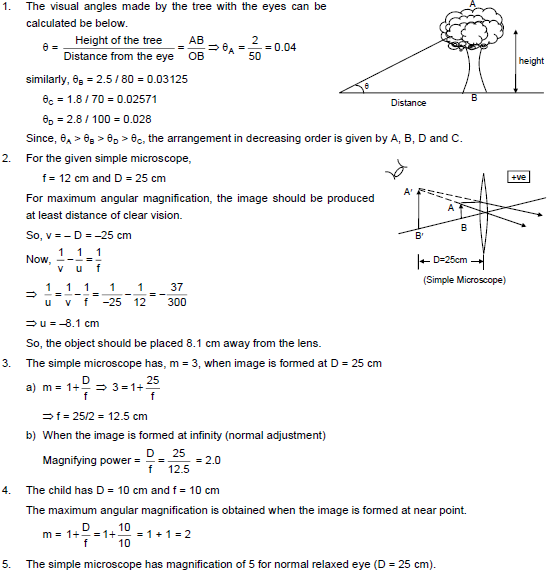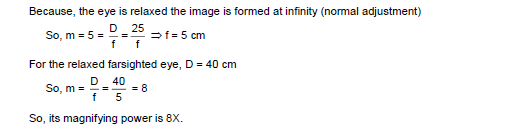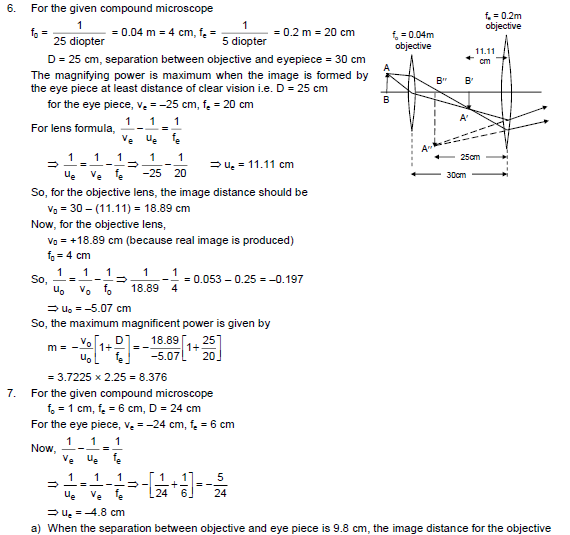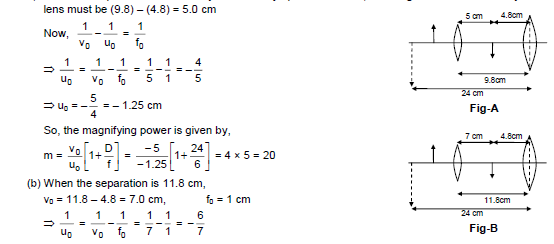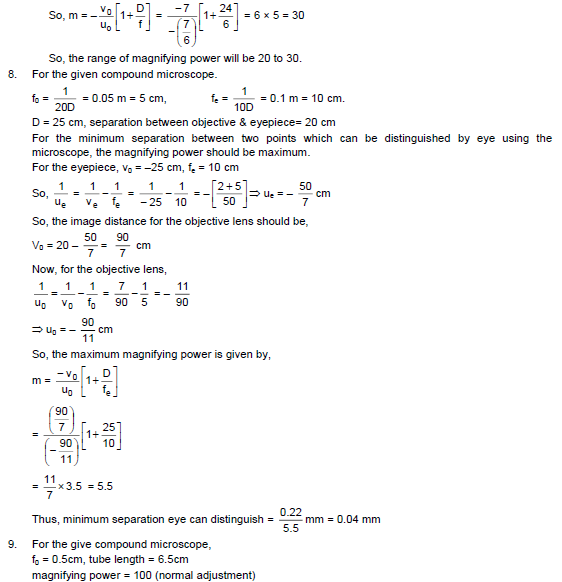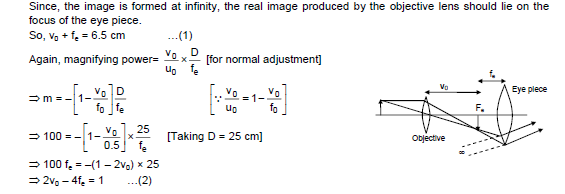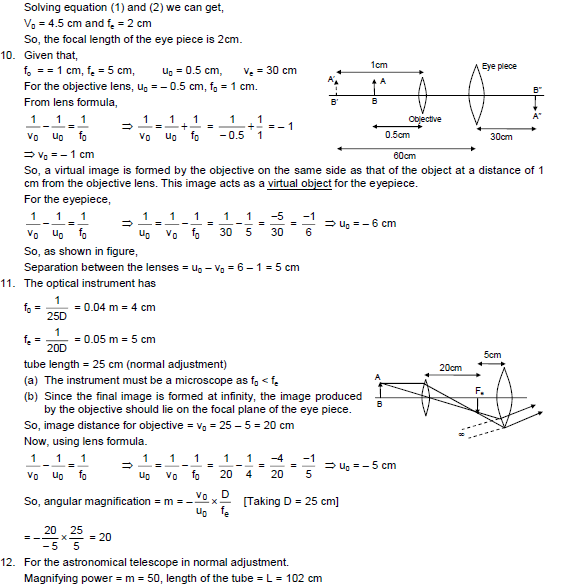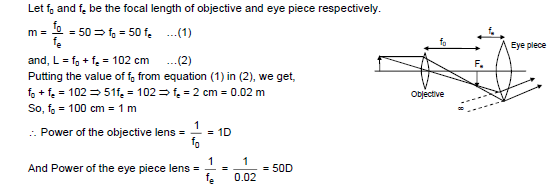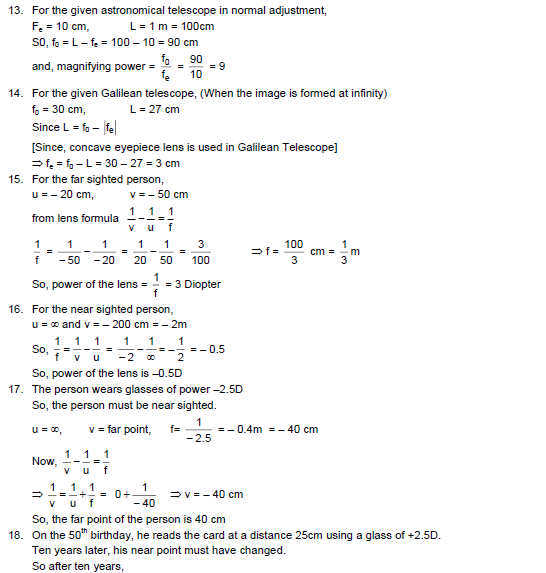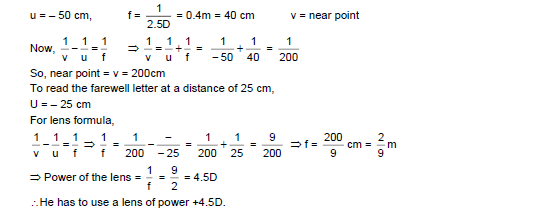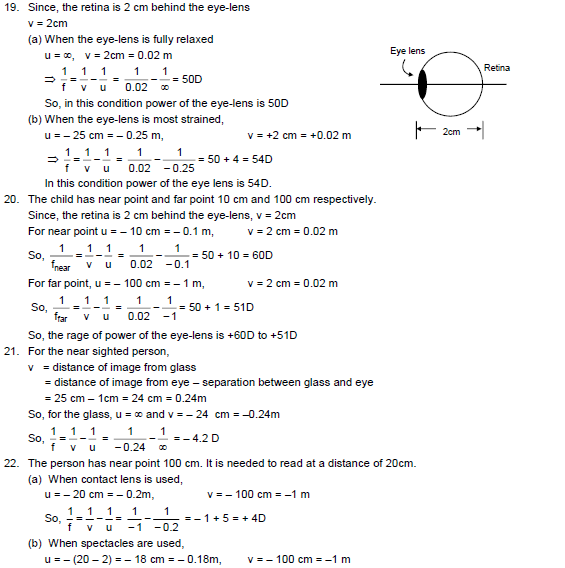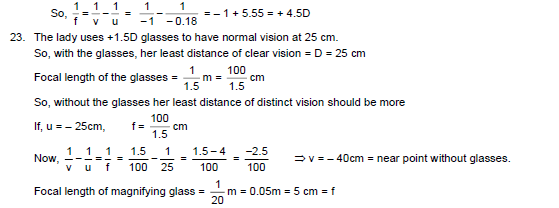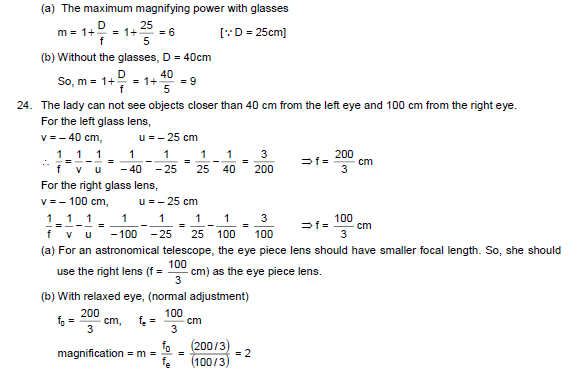# HC Verma Solutions Vol 1 Chapter 19 Optical Instruments

HC Verma Solutions Vol 1 Chapter 19 Optical Instruments can be used as a productive reference tool while preparing for various competitive exams. It offers students the right answers and methods to solve questions given in this chapter. There are questions related to visual angles, maximum angular magnification and the distance of the object from the lens to produce an image, etc. Students will also be solving problems such as:

• Questions related to finding the magnifying power of the simple microscope when images are formed at various points.
• Problems based on lens formula and the image and object distance from the objective lens.
• The difference in image distance when the objective and the eyepiece of a compound microscope is adjusted to different ranges.
• Questions on angular magnification when adjustments are made to the optical instrument whether it would be an astronomical telescope or Galilean telescope, etc.
• Students will deal with questions on near and farsightedness and the kind of lens that is used for both including the ranges of the power of the eye lens.

Students will find the solutions given below. The can also download the HC Verma solutions PDF from the link provided below.

Students can further explore and get details about topics such as;

1. The Eye
2. The Apparent Size
3. Simple Microscope
4. Compound Microscope
5. Telescopes
6. Resolving Power of a Microscope and a Telescope
7. Defects of Vision

## Important Question In Chapter 19

1. Usually, when we talk about microscopes and telescopes the magnification properties are defined in terms of the ratios of angles? Can you give reasons as to why factors like the ratio of sizes of objects and sizes are not considered?

2. Will an image be inverted if we say that the angular magnification of a system is less than one?

3. It is through the eyes that we see different objects at certain distances. During this phenomenon what factors usually remain constant?

(a) image-distance from the eye-lens (b) object-distance from the eye-lens (c) focal length of the eye-lens (d) radii of curvature of the eye lens

4. What following factor should be increased to increase the angular magnification of a simple microscope?

(a) the aperture of the lens (b) the focal length of the lens (c) the power of the lens (d) object size

5. Let us place an object at a distance x under a microscope with a focal length f. What will the angular magnification that is formed depend upon?

(a) on f (b) on x (c) both f and x (d) none of them.

## HC Verma Solutions Vol 1 Geometrical Optics Chapter 19